# Website Development Introduction to Java Script clientside programming

• Slides: 32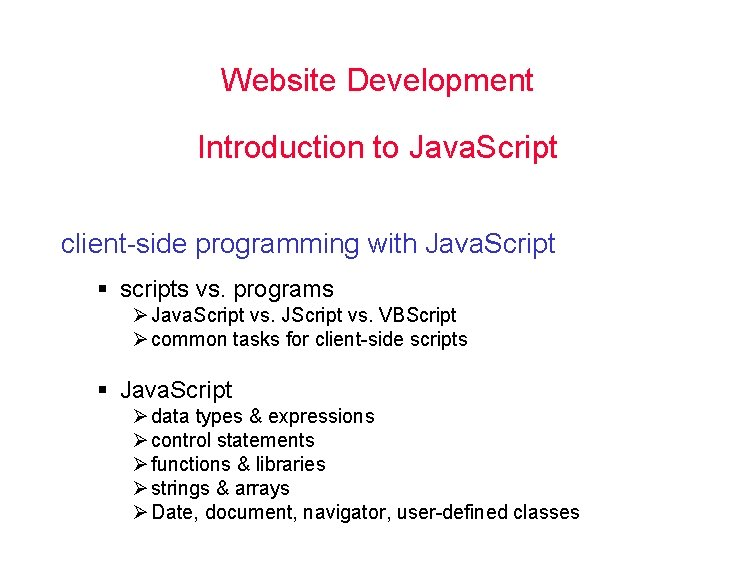Website Development Introduction to Java. Script client-side programming with Java. Script § scripts vs. programs Ø Java. Script vs. JScript vs. VBScript Ø common tasks for client-side scripts § Java. Script Ø data types & expressions Ø control statements Ø functions & libraries Ø strings & arrays Ø Date, document, navigator, user-defined classes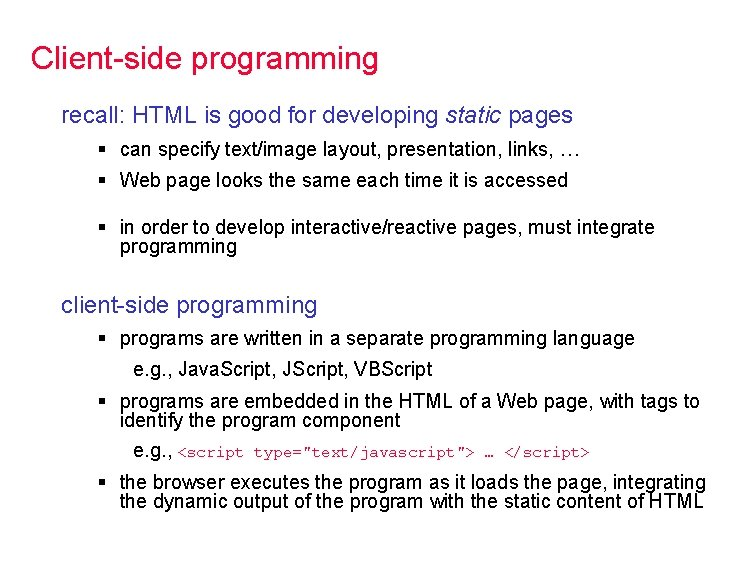Client-side programming recall: HTML is good for developing static pages § can specify text/image layout, presentation, links, … § Web page looks the same each time it is accessed § in order to develop interactive/reactive pages, must integrate programming client-side programming § programs are written in a separate programming language e. g. , Java. Script, JScript, VBScript § programs are embedded in the HTML of a Web page, with tags to identify the program component e. g. , <script type="text/javascript"> … </script> § the browser executes the program as it loads the page, integrating the dynamic output of the program with the static content of HTML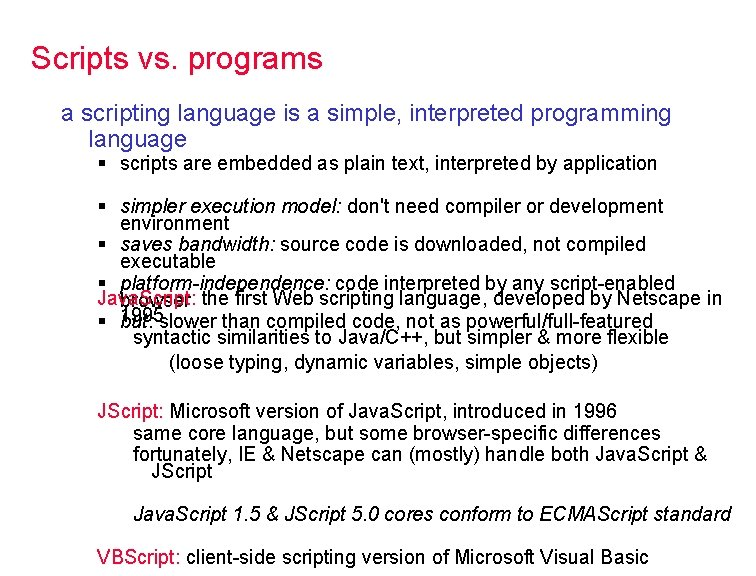Scripts vs. programs a scripting language is a simple, interpreted programming language § scripts are embedded as plain text, interpreted by application § simpler execution model: don't need compiler or development environment § saves bandwidth: source code is downloaded, not compiled executable § platform-independence: code interpreted by any script-enabled Java. Script: browser the first Web scripting language, developed by Netscape in § 1995 but: slower than compiled code, not as powerful/full-featured syntactic similarities to Java/C++, but simpler & more flexible (loose typing, dynamic variables, simple objects) JScript: Microsoft version of Java. Script, introduced in 1996 same core language, but some browser-specific differences fortunately, IE & Netscape can (mostly) handle both Java. Script & JScript Java. Script 1. 5 & JScript 5. 0 cores conform to ECMAScript standard VBScript: client-side scripting version of Microsoft Visual Basic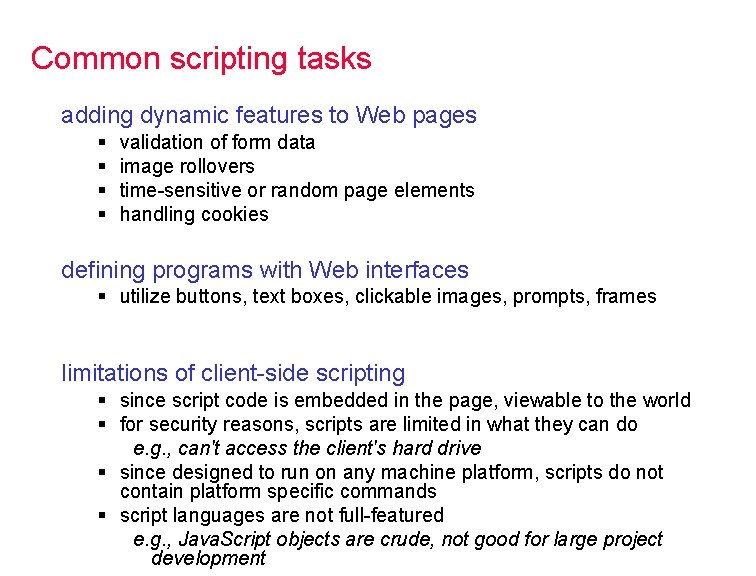Common scripting tasks adding dynamic features to Web pages § § validation of form data image rollovers time-sensitive or random page elements handling cookies defining programs with Web interfaces § utilize buttons, text boxes, clickable images, prompts, frames limitations of client-side scripting § since script code is embedded in the page, viewable to the world § for security reasons, scripts are limited in what they can do e. g. , can't access the client's hard drive § since designed to run on any machine platform, scripts do not contain platform specific commands § script languages are not full-featured e. g. , Java. Script objects are crude, not good for large project developmentJava. Script code can be embedded in a Web page using SCRIPT tags § the output of Java. Script code is displayed as if directly entered in <html> HTML <!-- js 01. html --> <head> <title>Java. Script Page</title> </head> <body> <script type="text/javascript"> // silly code to demonstrate output document. write displays text in page text to be displayed can include HTML tags the tags are interpreted by the browser when the text is displayed document. write("Hello world!"); document. write("<p>How are " + "<i>you</i>? </p>"); </script> <p>Here is some static text as well. </p> </body> </html> as in C++/Java, statements end with ; Java. Script comments similar to C++/Java starts a single line comment //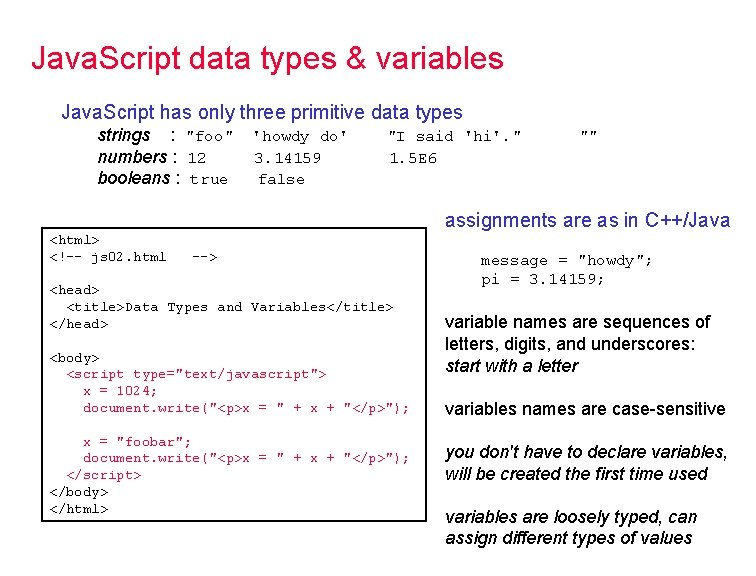Java. Script data types & variables Java. Script has only three primitive data types strings : "foo" numbers : 12 booleans : true 'howdy do' 3. 14159 false "I said 'hi'. " 1. 5 E 6 "" assignments are as in C++/Java <html> <!-- js 02. html --> <head> <title>Data Types and Variables</title> </head> <body> <script type="text/javascript"> x = 1024; document. write("<p>x = " + x + "</p>"); x = "foobar"; document. write("<p>x = " + x + "</p>"); </script> </body> </html> message = "howdy"; pi = 3. 14159; variable names are sequences of letters, digits, and underscores: start with a letter variables names are case-sensitive you don't have to declare variables, will be created the first time used variables are loosely typed, can assign different types of valuesJava. Script operators & expressions standard C++/Java operators are provided in Java. Script numeric : strings: relational: logical : <html> <!-- js 03. html + - * / % (remainder) + (concatenation) == != < <= > >= && || ! --> <head> <title>Operators and Expressions</title> </head> <body> <script type="text/javascript"> x = 5; document. write("x+1 = " + x+1 + " "); document. write(x + 1 + " = x+1 "); document. write("x+1 = " + (x+1)); </script> </body> </html> as in C++/Java, precedence rules apply to expressions (* / %) (+ -) (&& || !) operators are left-associative (evaluated in left-to-right order) must be careful when mixing strings and numbers number + number addition string + string concatenation string + number convert number to string, then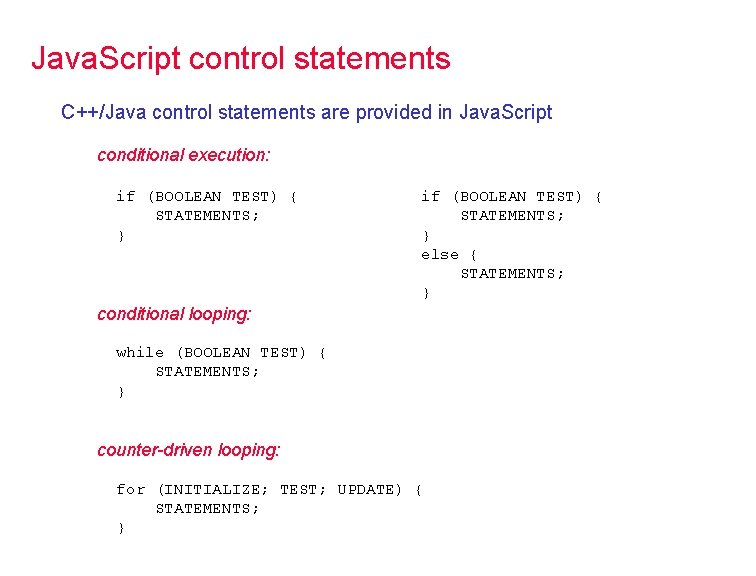Java. Script control statements C++/Java control statements are provided in Java. Script conditional execution: if (BOOLEAN TEST) { STATEMENTS; } else { STATEMENTS; } conditional looping: while (BOOLEAN TEST) { STATEMENTS; } counter-driven looping: for (INITIALIZE; TEST; UPDATE) { STATEMENTS; }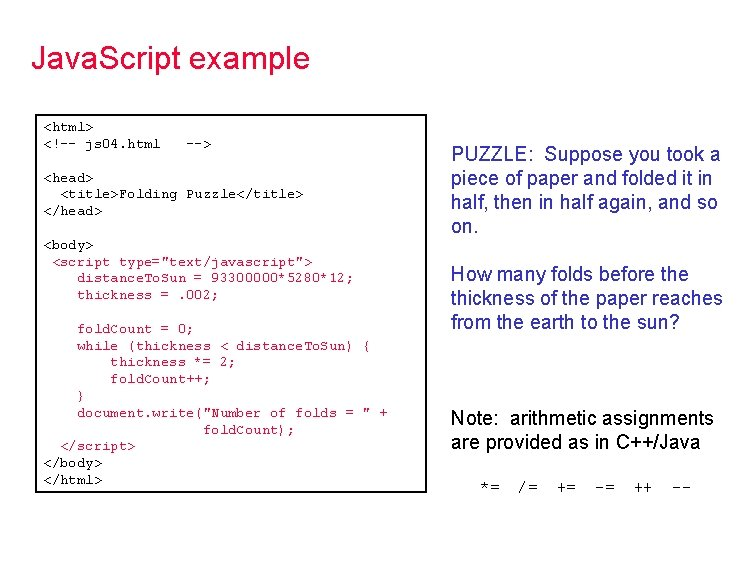Java. Script example <html> <!-- js 04. html --> <head> <title>Folding Puzzle</title> </head> <body> <script type="text/javascript"> distance. To. Sun = 93300000*5280*12; thickness =. 002; fold. Count = 0; while (thickness < distance. To. Sun) { thickness *= 2; fold. Count++; } document. write("Number of folds = " + fold. Count); </script> </body> </html> PUZZLE: Suppose you took a piece of paper and folded it in half, then in half again, and so on. How many folds before thickness of the paper reaches from the earth to the sun? Note: arithmetic assignments are provided as in C++/Java *= /= += -= ++ --Java. Script Math routines <html> <!-- js 05. html --> <head> <title>Mystery Program</title> </head> <body> <script type="text/javascript"> max. Range = 100; for(i = 1; i <= max. Range; i++) { if (Math. pow(Math. floor(Math. sqrt(i)), 2) == i) { document. write(i + " "); } } </script> </body> </html> the predefined Math object contains routines and constants Math. sqrt Math. pow Math. abs Math. max Math. min Math. floor Math. ceil Math. round Math. PI Math. E QUESTION: what does this program do?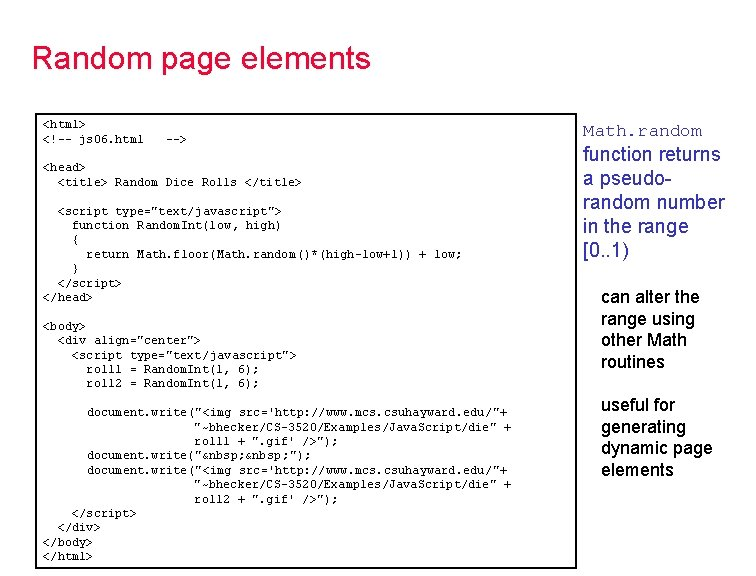Random page elements <html> <!-- js 06. html --> <head> <title> Random Dice Rolls </title> <script type="text/javascript"> function Random. Int(low, high) { return Math. floor(Math. random()*(high-low+1)) + low; } </script> </head> <body> <div align="center"> <script type="text/javascript"> roll 1 = Random. Int(1, 6); roll 2 = Random. Int(1, 6); document. write("<img src='http: //www. mcs. csuhayward. edu/"+ "~bhecker/CS-3520/Examples/Java. Script/die" + roll 1 + ". gif' />"); document. write("  &nbsp ; "); document. write("<img src='http: //www. mcs. csuhayward. edu/"+ "~bhecker/CS-3520/Examples/Java. Script/die" + roll 2 + ". gif' />"); </script> </div> </body> </html> Math. random function returns a pseudorandom number in the range [0. . 1) can alter the range using other Math routines useful for generating dynamic page elements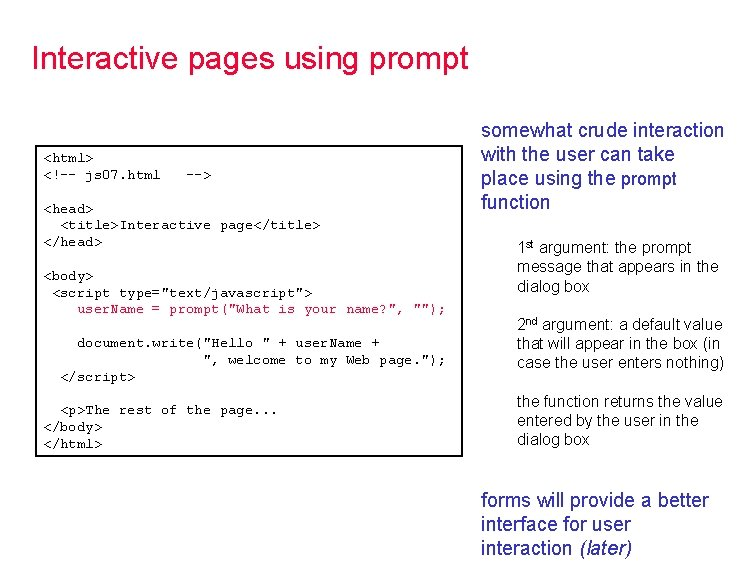Interactive pages using prompt <html> <!-- js 07. html --> <head> <title>Interactive page</title> </head> <body> <script type="text/javascript"> user. Name = prompt("What is your name? ", ""); document. write("Hello " + user. Name + ", welcome to my Web page. "); </script> <p>The rest of the page. . . </body> </html> somewhat crude interaction with the user can take place using the prompt function 1 st argument: the prompt message that appears in the dialog box 2 nd argument: a default value that will appear in the box (in case the user enters nothing) the function returns the value entered by the user in the dialog box forms will provide a better interface for user interaction (later)Prompting for numbers <html> <!-- js 08. html --> <head> <title>Prompting for numbers</title> </head> <body> <script type="text/javascript"> num 1 = prompt("Enter the first number", "1"); num 1 = parse. Float(num 1); num 2 = prompt("Enter the second number", "2"); num 2 = parse. Float(num 2); document. write("The sum of the numbers is " + (num 1 + num 2)); </script> </body> </html> Note: prompt always returns a string if the user enters the number 12 at the prompt, the string "12" is returned recall: + applied to strings gives concatenation if numbers are to be read using prompt, they must be explicitly converted to numbers using parse. Float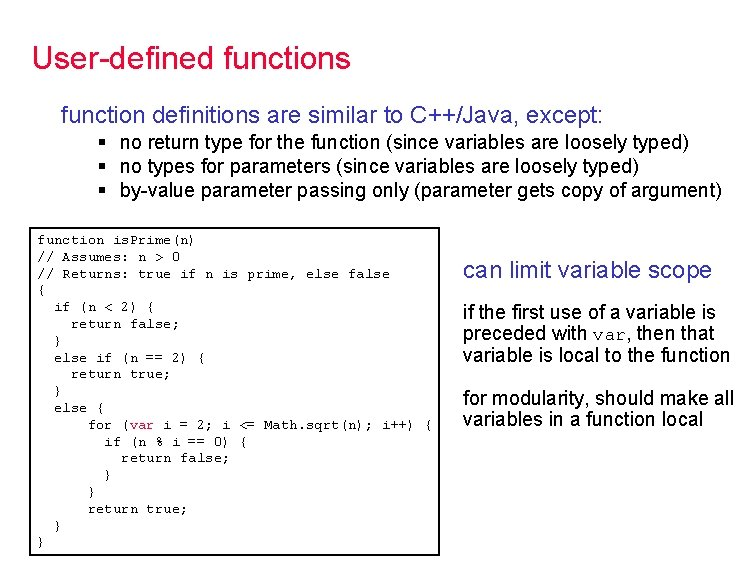User-defined functions function definitions are similar to C++/Java, except: § no return type for the function (since variables are loosely typed) § no types for parameters (since variables are loosely typed) § by-value parameter passing only (parameter gets copy of argument) function is. Prime(n) // Assumes: n > 0 // Returns: true if n is prime, else false { if (n < 2) { return false; } else if (n == 2) { return true; } else { for (var i = 2; i <= Math. sqrt(n); i++) { if (n % i == 0) { return false; } } return true; } } can limit variable scope if the first use of a variable is preceded with var, then that variable is local to the function for modularity, should make all variables in a function local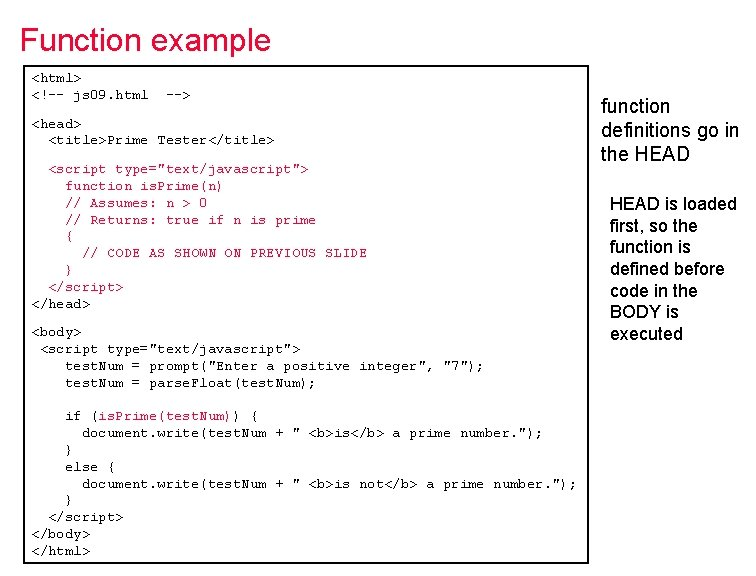Function example <html> <!-- js 09. html --> <head> <title>Prime Tester</title> <script type="text/javascript"> function is. Prime(n) // Assumes: n > 0 // Returns: true if n is prime { // CODE AS SHOWN ON PREVIOUS SLIDE } </script> </head> <body> <script type="text/javascript"> test. Num = prompt("Enter a positive integer", "7"); test. Num = parse. Float(test. Num); if (is. Prime(test. Num)) { document. write(test. Num + " <b>is</b> a prime number. "); } else { document. write(test. Num + " <b>is not</b> a prime number. "); } </script> </body> </html> function definitions go in the HEAD is loaded first, so the function is defined before code in the BODY is executed<html> <!-- js 10. html --> <head> <title> Random Dice Rolls Revisited</title> <script type="text/javascript"> function Random. Int(low, high) // Assumes: low <= high // Returns: random integer in range [low. . high] { return Math. floor(Math. random()*(high-low+1)) + low; } </script> </head> <body> <div align="center"> <script type="text/javascript"> roll 1 = Random. Int(1, 6); roll 2 = Random. Int(1, 6); document. write("<img src='http: //www. csuhayward. edu/"+ "~bhecker/cs-3520/Images/die" + roll 1 + ". gif' />"); document. write("  "); document. write("<img src='http: //www. csuhayard. edu/"+ "~bhecker/cs-3520/Images/die" + roll 2 + ". gif' />"); </script> </div> </body> </html> Another example recall the dynamic dice page could define a function for generating random numbers in a range, then use whenever needed easier to remember, promotes reuseJava. Script libraries better still: if you define functions that may be useful to many pages, store in a separate library file and load the library when needed the file at http: //www. csuhayward. edu/~bhecker/3520/Java. Script/random. js contains definitions of the following functions: Random. Num(low, high) returns random real in range [low. . high) Random. Int(low, high) returns random integer in range [low. . high) Random. Char(string) returns random character from the string Random. One. Of([item 1, …, item. N]) returns random item from list/array Note: as with external style sheets, no tags in the Java. Script library file load a library using the SRC attribute in the SCRIPT tag (nothing between the tags) <script type="text/javascript" src="http: //www. csuhayward. edu/~bhecker/cs 3520/Java. Script/random. js"> </script>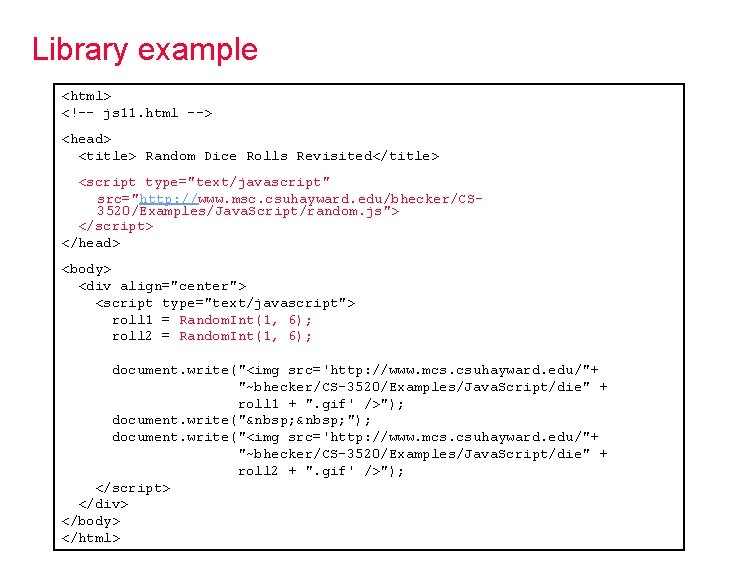Library example <html> <!-- js 11. html --> <head> <title> Random Dice Rolls Revisited</title> <script type="text/javascript" src="http: //www. msc. csuhayward. edu/bhecker/CS 3520/Examples/Java. Script/random. js"> </script> </head> <body> <div align="center"> <script type="text/javascript"> roll 1 = Random. Int(1, 6); roll 2 = Random. Int(1, 6); document. write("<img src='http: //www. mcs. csuhayward. edu/"+ "~bhecker/CS-3520/Examples/Java. Script/die" + roll 1 + ". gif' />"); document. write("  "); document. write("<img src='http: //www. mcs. csuhayward. edu/"+ "~bhecker/CS-3520/Examples/Java. Script/die" + roll 2 + ". gif' />"); </script> </div> </body> </html>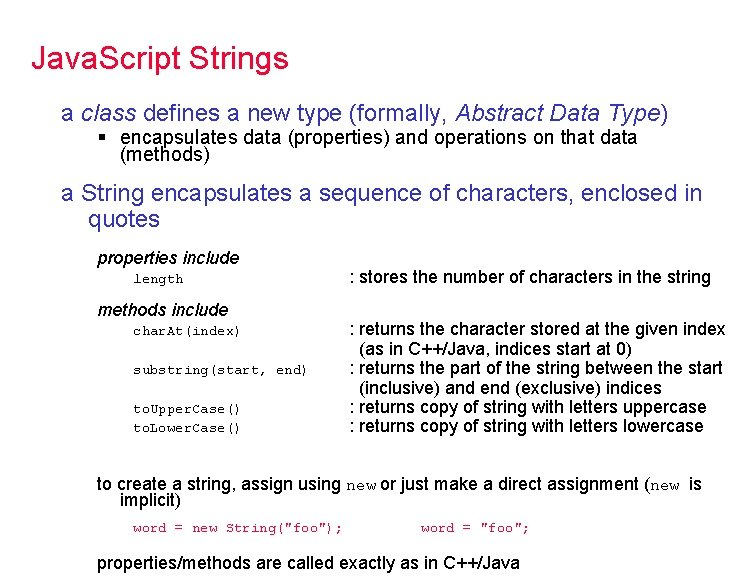Java. Script Strings a class defines a new type (formally, Abstract Data Type) § encapsulates data (properties) and operations on that data (methods) a String encapsulates a sequence of characters, enclosed in quotes properties include length methods include char. At(index) substring(start, end) to. Upper. Case() to. Lower. Case() : stores the number of characters in the string : returns the character stored at the given index (as in C++/Java, indices start at 0) : returns the part of the string between the start (inclusive) and end (exclusive) indices : returns copy of string with letters uppercase : returns copy of string with letters lowercase to create a string, assign using new or just make a direct assignment (new is implicit) word = new String("foo"); word = "foo"; properties/methods are called exactly as in C++/Java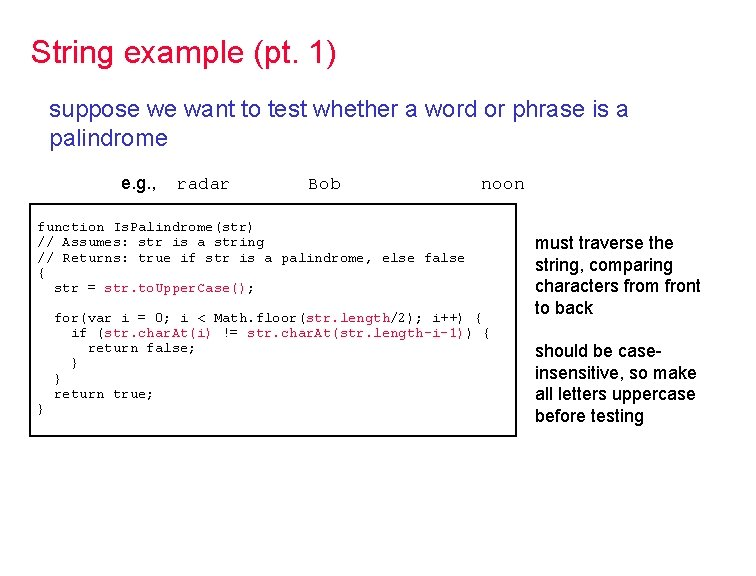String example (pt. 1) suppose we want to test whether a word or phrase is a palindrome e. g. , radar Bob noon function Is. Palindrome(str) // Assumes: str is a string // Returns: true if str is a palindrome, else false { str = str. to. Upper. Case(); } for(var i = 0; i < Math. floor(str. length/2); i++) { if (str. char. At(i) != str. char. At(str. length-i-1)) { return false; } } return true; must traverse the string, comparing characters from front to back should be caseinsensitive, so make all letters uppercase before testingString example (pt. 2) function Strip(str) // Assumes: str is a string // Returns: str with all but capital letters removed { var copy = ""; for (var i = 0; i < str. length; i++) { if (str. char. At(i) >= "A" && str. char. At(i) <= "Z") { copy += str. char. At(i); } } return copy; } function Is. Palindrome(str) // Assumes: str is a string // Returns: true if str is a palindrome, else false { str = Strip(str. to. Upper. Case()); } for(var i = 0; i < Math. floor(str. length/2); i++) { if (str. char. At(i) != str. char. At(str. length-i-1)) { return false; } } return true; better yet, we would like to be able to test phrases Madam, I'm Adam. A man, a plan, a canal: Panama! must strip non-letters out of the phrase, then test as before to handle phrases, must be able to strip out nonletters<html> <!-- js 12. html --> <head> <title>Palindrome Checker</title> <script type="text/javascript"> function Strip(str) { // CODE AS SHOWN ON PREVIOUS SLIDE } function Is. Palindrome(str) { // CODE AS SHOWN ON PREVIOUS SLIDE } </script> </head> <body> <script type="text/javascript"> text = prompt("Enter a word or phrase", "Madam, I'm Adam"); if (Is. Palindrome(text)) { document. write("'" + text + "' <b>is</b> a palindrome. "); } else { document. write("'" + text + "' <b>is not</b> a palindrome. "); } </script> </body> </html>Java. Script arrays store a sequence of items, accessible via an index since Java. Script is loosely typed, elements do not have to be the same type § to create an array, allocate space using new (or can assign directly) items = new Array(10); // allocates space for 10 items = new Array(); // if no size, will adjust dynamically items = [0, 0, 0, 0]; // can assign size & values [] § to access an array element, use [] (as in C++/Java) for (i = 0; i < 10; i++) { items[i] = 0; // stores 0 at each index } § the length property stores the number of items in the array for (i = 0; i < items. length; i++) { document. write(items[i] + " "); } // displays elements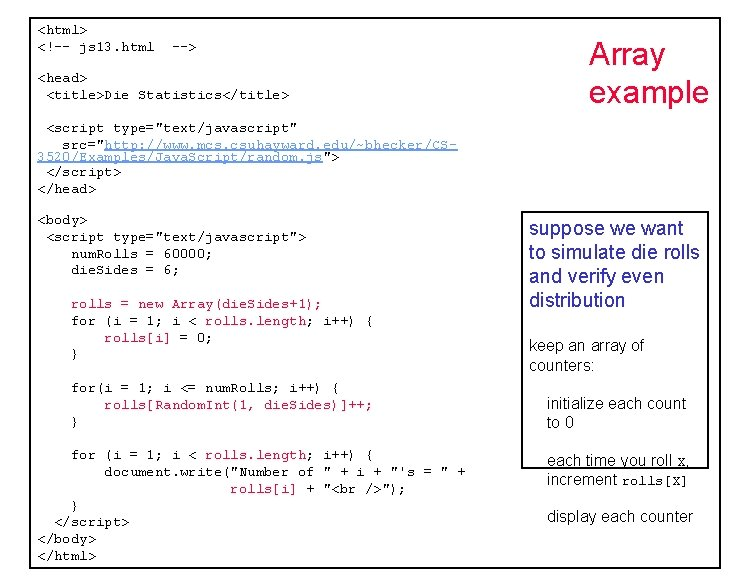<html> <!-- js 13. html --> <head> <title>Die Statistics</title> Array example <script type="text/javascript" src="http: //www. mcs. csuhayward. edu/~bhecker/CS 3520/Examples/Java. Script/random. js"> </script> </head> <body> <script type="text/javascript"> num. Rolls = 60000; die. Sides = 6; rolls = new Array(die. Sides+1); for (i = 1; i < rolls. length; i++) { rolls[i] = 0; } for(i = 1; i <= num. Rolls; i++) { rolls[Random. Int(1, die. Sides)]++; } for (i = 1; i < rolls. length; i++) { document. write("Number of " + i + "'s = " + rolls[i] + " "); } </script> </body> </html> suppose we want to simulate die rolls and verify even distribution keep an array of counters: initialize each count to 0 each time you roll X, increment rolls[X] display each counter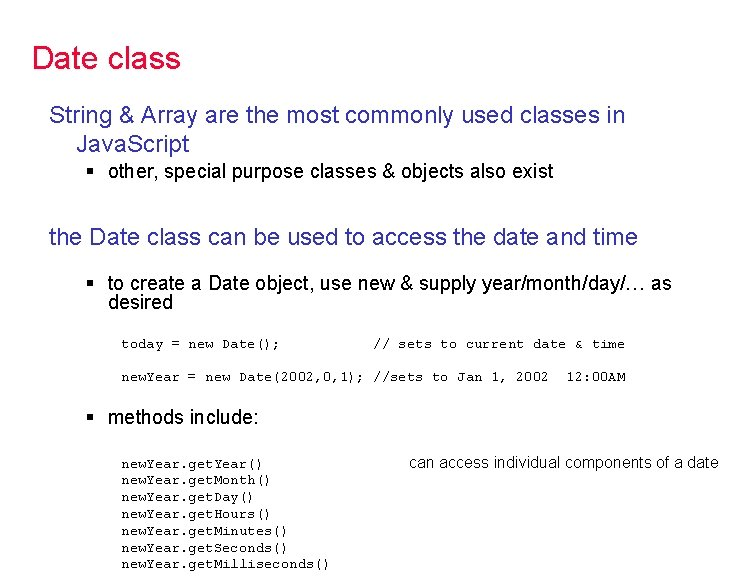Date class String & Array are the most commonly used classes in Java. Script § other, special purpose classes & objects also exist the Date class can be used to access the date and time § to create a Date object, use new & supply year/month/day/… as desired today = new Date(); // sets to current date & time new. Year = new Date(2002, 0, 1); //sets to Jan 1, 2002 12: 00 AM § methods include: new. Year. get. Year() new. Year. get. Month() new. Year. get. Day() new. Year. get. Hours() new. Year. get. Minutes() new. Year. get. Seconds() new. Year. get. Milliseconds() can access individual components of a date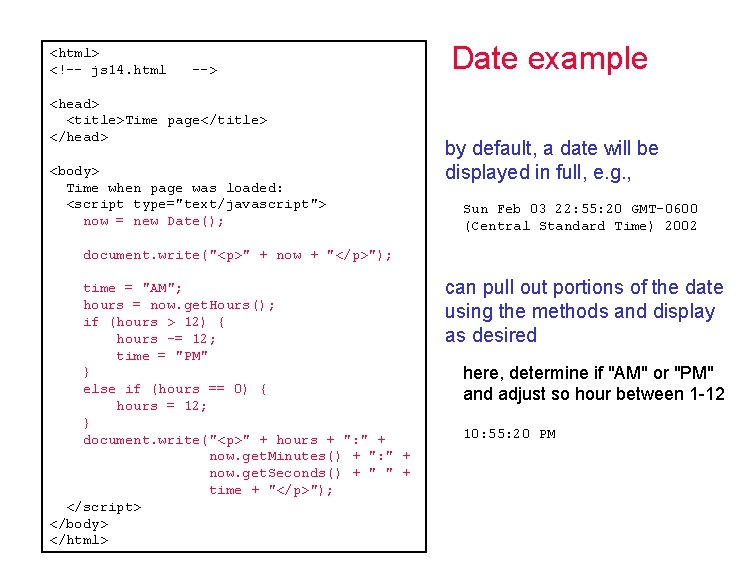<html> <!-- js 14. html --> <head> <title>Time page</title> </head> <body> Time when page was loaded: <script type="text/javascript"> now = new Date(); Date example by default, a date will be displayed in full, e. g. , Sun Feb 03 22: 55: 20 GMT-0600 (Central Standard Time) 2002 document. write("<p>" + now + "</p>"); time = "AM"; hours = now. get. Hours(); if (hours > 12) { hours -= 12; time = "PM" } else if (hours == 0) { hours = 12; } document. write("<p>" + hours + ": " + now. get. Minutes() + ": " + now. get. Seconds() + " " + time + "</p>"); </script> </body> </html> can pull out portions of the date using the methods and display as desired here, determine if "AM" or "PM" and adjust so hour between 1 -12 10: 55: 20 PM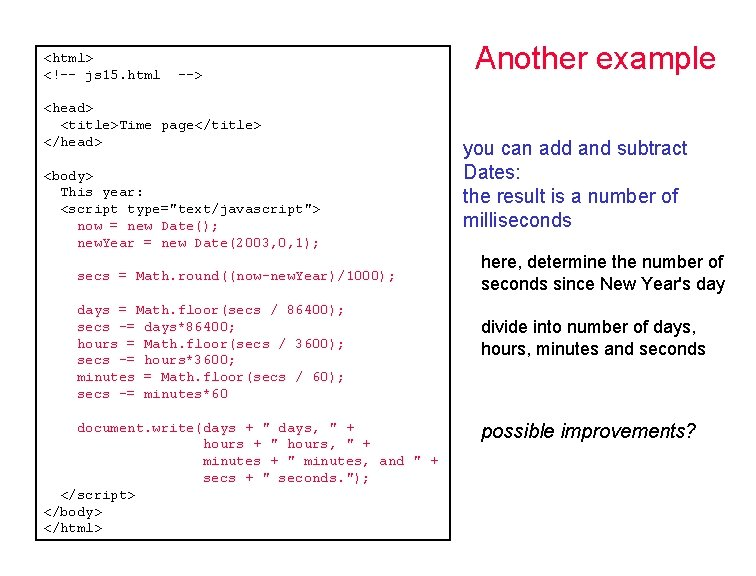<html> <!-- js 15. html --> <head> <title>Time page</title> </head> <body> This year: <script type="text/javascript"> now = new Date(); new. Year = new Date(2003, 0, 1); secs = Math. round((now-new. Year)/1000); days = Math. floor(secs / 86400); secs -= days*86400; hours = Math. floor(secs / 3600); secs -= hours*3600; minutes = Math. floor(secs / 60); secs -= minutes*60 document. write(days + " days, " + hours + " hours, " + minutes + " minutes, and " + secs + " seconds. "); </script> </body> </html> Another example you can add and subtract Dates: the result is a number of milliseconds here, determine the number of seconds since New Year's day divide into number of days, hours, minutes and seconds possible improvements?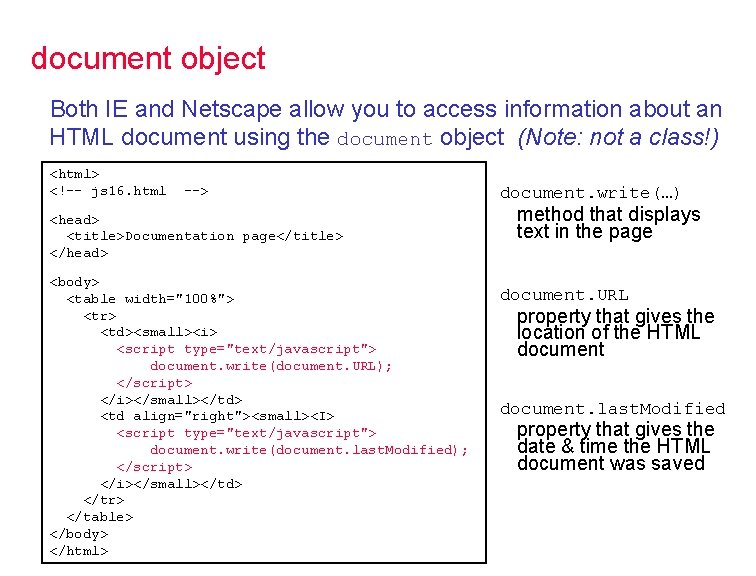document object Both IE and Netscape allow you to access information about an HTML document using the document object (Note: not a class!) <html> <!-- js 16. html --> <head> <title>Documentation page</title> </head> <body> <table width="100%"> <tr> <td><small><i> <script type="text/javascript"> document. write(document. URL); </script> </i></small></td> <td align="right"><small><I> <script type="text/javascript"> document. write(document. last. Modified); </script> </i></small></td> </tr> </table> </body> </html> document. write(…) method that displays text in the page document. URL property that gives the location of the HTML document. last. Modified property that gives the date & time the HTML document was savednavigator object navigator. app. Name property that gives the browser name navigator. app. Version property that gives the browser version <!-- MSIE. css --> a {text-decoration: none; font-size: larger; color: red; font-family: Arial} a: hover {color: blue} <!-- Netscape. css --> a {font-family: Arial; color: white; background-color: red} <html> <!-- js 17. html --> <head> <title>Dynamic Style Page</title> <script type="text/javascript"> if (navigator. app. Name == "Netscape") { document. write('<link rel=stylesheet '+ 'type="text/css" href="Netscape. css">'); } else { document. write('<link rel=stylesheet ' + 'type="text/css" href="MSIE. css">'); } </script> </head> <body> Here is some text with a <a href="javascript: alert('GO AWAY')">link</a>. </body> </html>User-defined classes can define new classes, but the notation is awkward § simply define a function that serves as a constructor § specify data fields & methods using this § no data hiding: can't protect data or methods // Die. js // // Die class definition ////////////////////// function Die(sides) { this. num. Sides = sides; this. num. Rolls = 0; this. Roll = Roll; } function Roll() { this. num. Rolls++; return Math. floor(Math. random()*this. num. Sides) + 1; } define Die function (i. e. , constructor) initialize data fields in the function, preceded with this similarly, assign method to separately defined function (which uses this to access data)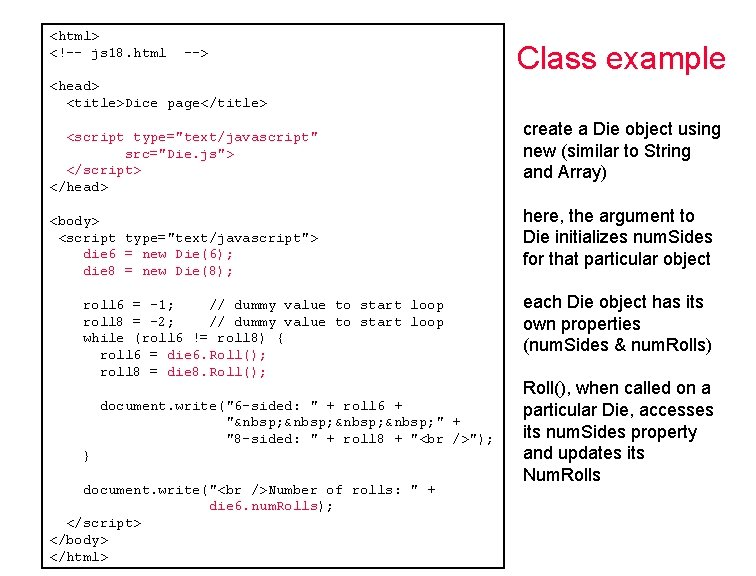<html> <!-- js 18. html --> Class example <head> <title>Dice page</title> <script type="text/javascript" src="Die. js"> </script> </head> <body> <script type="text/javascript"> die 6 = new Die(6); die 8 = new Die(8); roll 6 = -1; // dummy value to start loop roll 8 = -2; // dummy value to start loop while (roll 6 != roll 8) { roll 6 = die 6. Roll(); roll 8 = die 8. Roll(); document. write("6 -sided: " + roll 6 + "  " + "8 -sided: " + roll 8 + " "); } document. write(" Number of rolls: " + die 6. num. Rolls); </script> </body> </html> create a Die object using new (similar to String and Array) here, the argument to Die initializes num. Sides for that particular object each Die object has its own properties (num. Sides & num. Rolls) Roll(), when called on a particular Die, accesses its num. Sides property and updates its Num. Rolls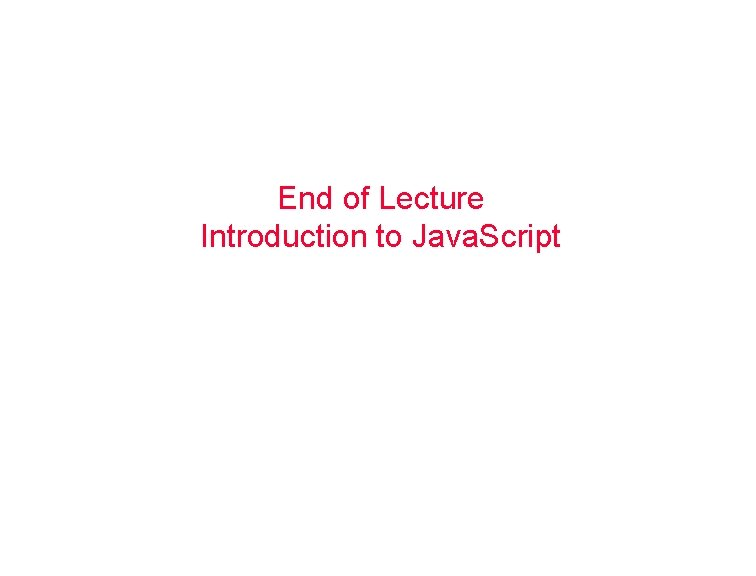End of Lecture Introduction to Java. Script